#### Study Material and Notes of Ch 10 Light – Refraction Class 10th Science

Topics in the Chapter

• Introduction
• Refraction
• Laws of Refraction
→ Snell's law
→ Refractive index
→ Absolute refractive index
→ Optically denser medium
→ Optically rarer medium
→ Spherical lens
• Rules for image formation by convex lens
• Ray diagram of image formed by Convex lens
→ When object is at infinity
→ When object is beyond 2F1
→ When object is at 2F1
→ When object is between F1 and 2F1
→ When object is at F1
→ When object is between F1 and optical centre
• Rules for image formation by concave lens
• Ray diagrams of images formed by a concave lens
→ When object is placed at infinity
→ When object is placed between infinity and optical centre
• Sign convention for spherical lens
→ Lens Formula
→ Magnification
• Power of a lens

Introduction

→ Refraction is bending of light when it enters obliquely from one transparent medium to another.

→ Speed of light is maximum in vaccum. It is 3 × 108 m/s.

→ Cause of refraction: Change in speed of light.

• Some examples of refraction

→ The bottom of swimming pool appears higher.
→ A pencil partially immersed in water appears to be bent at the interface of water and air.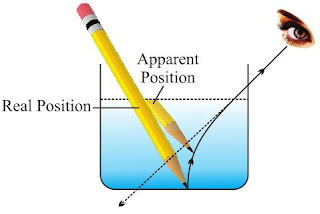→ Lemons placed in a glass tumbler appear bigger.
→ Letters of a book appear to be raised when seen through a glass slab.

Refraction

→ Bouncing back of light when it strikes on a polished surface like mirror.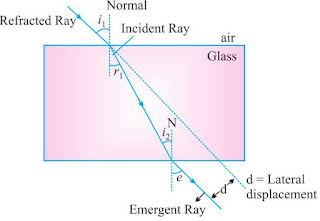→ The extent of bending of ray of light at the opposite parallel faces of rectangular glass slab is equal and opposite, so the ray emerges parallel to incident ray.

• Lateral displacement depends on :
→ Refractive index of glass slab
→ Thickness of the glass slab

Laws of Refraction

(i) The incident ray, the refracted ray and the normal to the interface of two transparent media at the point of incidence, all lie in the same plane.

(ii) Snell’s law: The ratio of sine of angle of incidence to the sine of angle of refraction is a constant, for a light of given colour and for a given pair of media.

sin i/sin r = constant

Refractive index (n): The ratio of speed of light in a given pair of media
n = Velocity of light in medium 1/Velocity of light in medium 2

→ n21 means refractive index of second medium with respect to first medium.
n21 = v1/v2

→ n12 means refractive index of second medium with respect to first medium.
n12 = v2/v1

Absolute Refractive Index: Refractive index of a medium with respect to vaccum or air.
n = (c/v) x c = 3 × 108 m/s.

→ Refractive index of one medium is reciprocal of other’s refractive index in a given pair.
n12 = 1/n21

→ If refractive index of medium 1 w.r.t. air is given as 1nair, and
If refractive index of medium 2 w.r.t. air is given as 2nair.
Then, refractive index of medium 1 w.r.t. medium 2 = (1nair)/(1nair)

→ Refractive index of diamond is the highest till date. It is 2.42. It means speed of light is 1/2.42 times less in diamond than in vaccum.

• Optically denser medium: Out of two given media, the medium with higher value of refractive index.

• Optically rarer medium: Out of two given media, the medium with lower value to refractive index.

→ When light enters obliquely from a rarer to a denser medium, it bends towards the normal.→ When light enters obliquely from denser to a rarer medium, it bends away from the normal.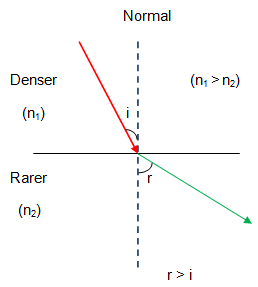→ Refractive index of a medium does not depend on physical density.

• Spherical lens: A transparent medium bound by two surfaces, of which one or both surfaces are curved.

 Convex Lens Concave Lens Thin from corners Thick from corners Thick at center Thin at centre Converging Diverbging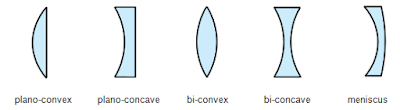Rules for image formation by convex lens

(i)  A ray of light parallel to principal axis of a convex lens always pass through the focus on the other side of the lens.(ii) A ray of light passing through the principal focus will emerge parallel to principal axis after refraction.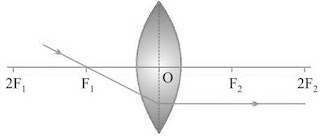(iii) A ray of light passing through the optical center will emerge without any deviation.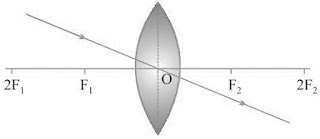Ray Diagrams of Imag formed by Convex Lens

(i) When object is at infinity
Image Position − At ‘F 2 ’
Nature of image – Real, inverted
Size – Point sized or highly diminished(ii) When object is beyond ‘2F1’
Image Position – Between ‘F2’ and ‘2F2’
Nature of image– Real, inverted
Size – Diminished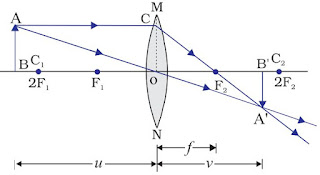(iii) When object is at ‘2F1 ’
Image Position – At ‘2F2 ’
Nature of image – Real, inverted
Size – Same size(iv) When object is between ‘F1’ and ‘2F1’
Image Position – Beyond ‘2F2’
Nature of image – Real, inverted
Size – Enlarged(v) When object is at ‘F1’
Image Position – At Infinity
Nature of image – Real, inverted
Size – Highly enlarged(vi) When object is between ‘F1’ and optical centre
Image Position – On the same side of the lens as object
Nature of image – Virtual and erect
Size – EnlargedRules for Image Formation by Concave Lens

(i)  A ray of light parallel to the principal axis appear to diverge from the principal focus located on the same side of the lens.(ii) A ray of light appearing to meet at the principal focus of a concave lens will emerge parallel to principal axis.(iii) A ray of light passing through the optical centre of a lens will emerge without any deviation.Ray Diagrams of Images Formed by a Concave Lens

(i) When object is placed at infinity
Image Position − At ‘F1’
Nature of image – Virtual, erect
Size – Point sized or highly diminished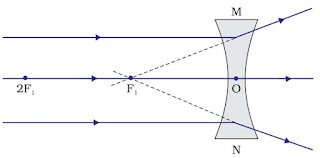(ii) When object is placed between infinity and optical centre
Image Position – Between ‘F’ and ‘O’
Nature of image – Virtual, erect
Size – DiminishedSign convention for spherical lenses

• Sign conventions are similar to the one used for spherical mirrors, except that measurements are taken from optical center of the lens.

• Focal length of convex lens = Positive
Focal length of concave lens = Negative

Lens Formula
1/v - 1/u = 1/f

Magnification
m = hi/h= v/u

Power of a lens

→ It is defined as the reciprocal of focal length in meter.

→ The degree of convergence or divergence of light rays is expressed in terms of power.

Power (P) = 1/v - 1/u = 1/f

→ SI unit of Power = dioptre = D

→ 1 D = 1 m-1

→ 1 dioptre is the power of lens whose focal length is one meter.

→ Power of convex lens = Positive

→ Power of concave lens = Negative

→ Power ∝ 1/(focal length or thickness)

→ Power of a lens combination (P) = P1 + P2 + P3 .........

Go Back to Class 10 Science Notes